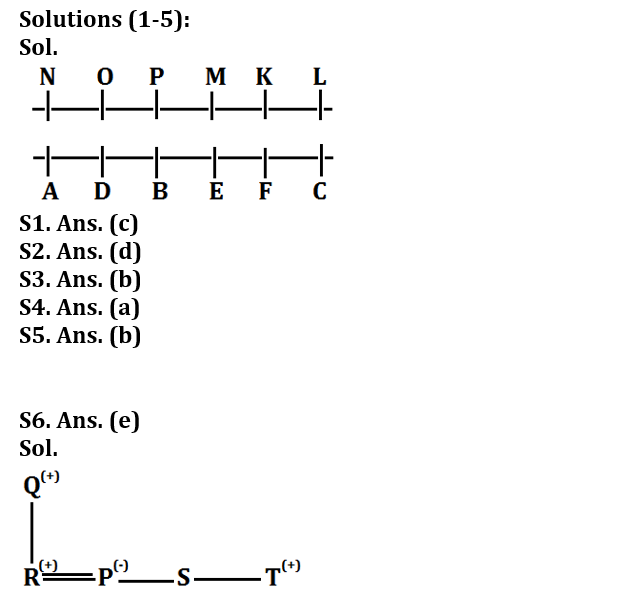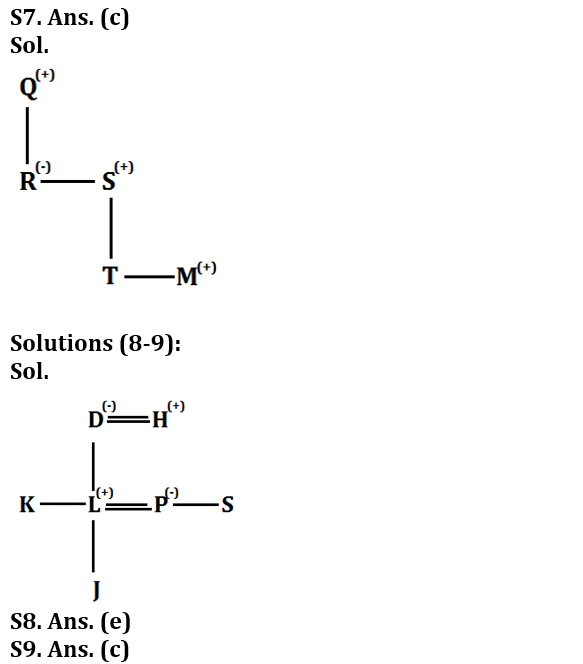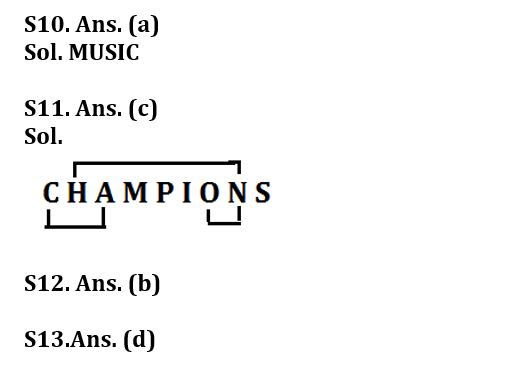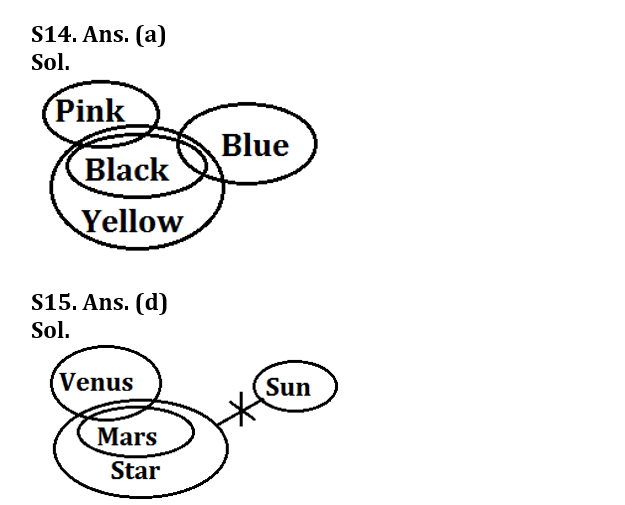Latest Banking jobs   »

# Reasoning Ability Quiz For Bank Foundation 2023- 28th January

Directions (1-5): Study the following information to answer the given questions:
Twelve people are sitting in two parallel rows containing six people each in such a way that there is an equal distance between adjacent persons. In row-1, K, L, M, N, O and P are sitting and all of them are facing South. In row-2, A, B, C, D, E and F are sitting and all of them are facing North. Therefore, in the given seating arrangement, each member of a row faces another member of the other row. C sits third to the right of B. B faces P and P does not sit at any of the extreme ends of the lines. N sits third to the right of M. F faces K. The one facing K sits third to the right of D. O and D does not sit at the extreme ends of the lines. A is not an immediate neighbour of B and P is not an immediate neighbour of N.

Q1. Who among the following sits opposite to M?
(a) D
(b) C
(c) E
(d) B
(e) None of these

Q2. How many persons sit between A and F?
(a) One
(b) Two
(c) More than Three
(d) Three
(e) None

Q3. Who among the following sits third to the left of P?
(a) O
(b) L
(c) K
(d) N
(e) None of these

Q4. What is the position of E with respect to D?
(a) Second to the right
(b) Immediate left
(c) Third to the right
(d) Second to the left
(e) None of these

Q5. Which among the following persons sit opposite to the one who sits second to the right of M?
(a) E
(b) D
(c) F
(d) C
(e) None of these

Directions (6 – 7): Study the following information and answer the questions given below:
‘Q + R’ means ‘Q is father of R’
‘Q ÷ R’ means ‘R is brother of Q’
‘Q × R’ means ‘Q is husband of R’
‘Q – R’ means ‘Q is sister of R’

Q6. In the expression “Q + R × P – S ÷ T” is true, then how is S related to R?
(a) Brother-in-law
(b) Sister-in-law
(c) Nephew
(d) Brother
(e) Can’t be determined

Q7. In the expression “Q + R – S + T ÷ M” is true, then how is M related to Q?
(a) Son
(b) Daughter
(c) Grandson
(d) Can’t be determined
(e) None of these

Directions (8-9): Study the following series carefully and answer the questions.
D is the mother of K and wife of H. L who is son of H, is married to P. P and S are siblings and D has one grandchild J. K is unmarried.

Q8. What is the relation of K with respect to J?
(a) Mother
(b) Aunt
(c) Brother
(d) Uncle
(e) Either (b) or (d)

Q9. How L is related to S?
(a) Mother-in-law
(b) Sister-in-law
(c) Brother-in-law
(d) Grandfather
(e) None of these

Q10. Which alphabet is 3rd from the left in the meaningful five-letter word formed from the 1st, 3rd, 5th, 9th and 11th letter of the word COMMUNITIES? If more than one word is formed, then mark answer as X and no meaningful word is formed then mark answer as Z.
(a) S
(b) U
(c) Z
(d) M
(e) X

Q11. How many pairs of letters are there in the word ‘CHAMPIONS’, each of which have as many letters between then in the word as they have between then in the English alphabet?
(a) One
(b) Two
(c) Three
(d) Four
(e) None

Q12. The position of how many alphabets will remain unchanged if each of the alphabets in the word ‘OVERCOME’ is arranged in alphabetical order from left to right?
(a) Four
(b) Two
(c) Three
(d) One
(e) None

Q13. What will come in place of the question mark (?) to make the expressions I < H as well as K ≥ J definitely true? J = Y ≤ I ? K < H
(a) ≥
(b) <
(c) >
(d) ≤
(e) None of these

Directions (14-15): In each of the questions below some statements are given followed by some conclusions. You have to take the given statements to be true even if they seem to be at variance with commonly known facts. Read all the conclusions and then decide which of the given conclusions logically follows from the given statements, disregarding commonly known facts. Give answer

Q14. Statements: Some Pink are Black.
All Black are Yellow.
Some Blue are Black.
Conclusions: I. Some Yellow are Pink.
II. Some Blue being Pink is a possibility.
III. Some Yellow are not Blue.
(a) Both I and II follows
(b) Either I or II follows
(c) Only III follows
(d) None follows
(e) Only I follows

Q15. Statements: Only a few Mars are Venus.
All Mars are Star.
No Sun is Star.
Conclusions: I. All Venus is star is a possibility
II. Some Venus is Sun
III. No Mars is Sun
(a) Only I follow
(b) Only II follows
(c) Only III follows
(d) Both I and III follows
(e) None of these

Solutions## FAQs

### What is the selection process of the Bank Clerk?

The selection process of the Bank Clerk is Prelims & Mains.

#### Congratulations!Union Budget 2023-24: Free PDF